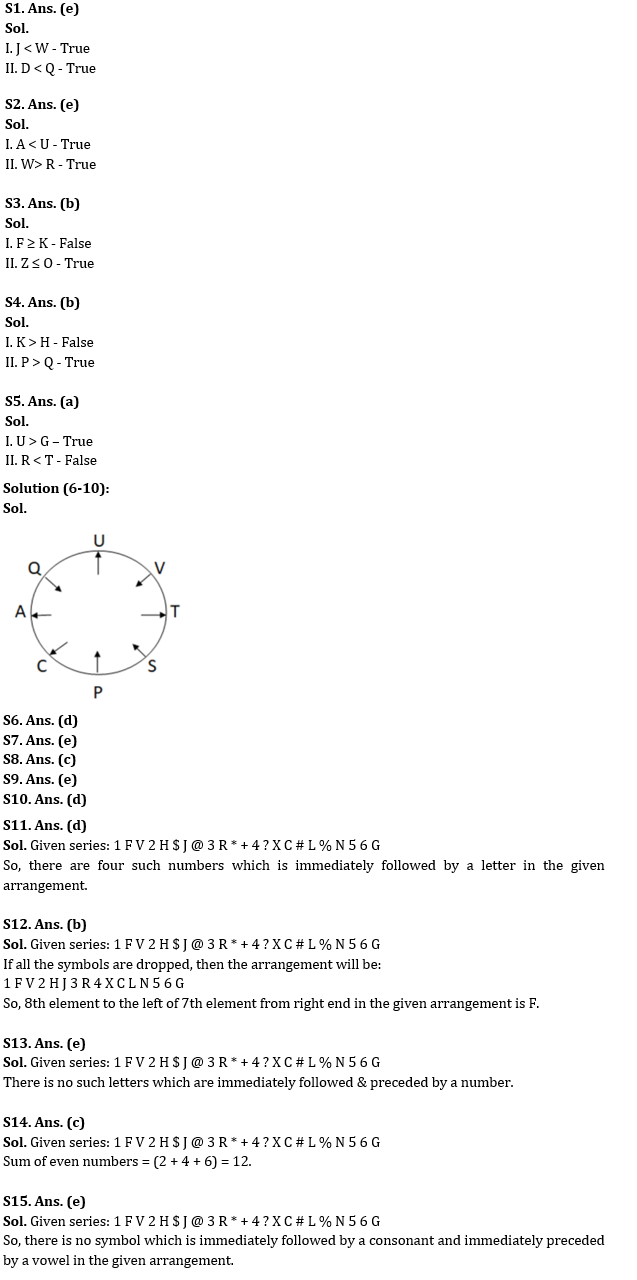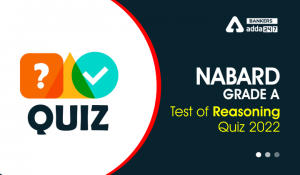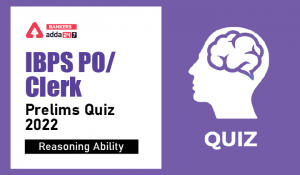Latest Banking jobs   »

# Reasoning Ability Quiz For IBPS RRB PO Clerk Prelims 2022- 8th July

Directions (1-5): In the following questions assuming the given statement to be true, find which of the conclusion(s) is/are definitely true and then give your answers accordingly.

Q1.
Statements:
J < C; A < W ≤ Q; D < O < W; D > C
Conclusions:
I. J < W
II. D < Q
(a) Only I is true
(b) Only II is true
(c) Either I or II is true
(d) Neither I nor II is true
(e) Both I and II are true

Q2.
Statements:
R ≤ D=E; A < Q = R; D < U < V; W > V
Conclusions:
I. A < U
II. W > R
(a) Only I is true
(b) Only II is true
(c) Either I or II is true
(d) Neither I nor II is true
(e) Both I and II are true

Q3.
Statements:
U< O=K; Z =D ≤ A ≤ F; D ≤ O
Conclusions:
I. F ≥ K
II. Z ≤ O
(a) Only I is true
(b) Only II is true
(c) Either I or II is true
(d) Neither I nor II is true
(e) Both I and II are true

Q4.
Statements:
K > Q ≤ U = H, T < P ≥ K < J
Conclusions:
I. K > H
II. P > Q
(a) Only I is true
(b) Only II is true
(c) Either I or II is true
(d) Neither I nor II is true
(e) Both I and II are true

Q5.
Statements:
G = H ≤ I < R, H < T = U
Conclusions:
I. U > G
II. R < T
(a) Only I is true
(b) Only II is true
(c) Either I or II is true
(d) Neither I nor II is true
(e) Both I and II are true

Directions (6-10): Study the following information carefully and answer the questions accordingly.
Eight people sit around a circular table. Some of them faces inside and some faces outside. S sits second to the left of V who doesn’t sit immediate right of T. Two people sit between S and A. Three people sit between P and U. C sits second to the right of Q who doesn’t sit immediate left of A. Q sits third to the left of P. The neighbors of A face the opposite direction to each other. S sits third to the right of U and face the opposite direction of T. Two people sit between C and U. Q faces inside.

Q6. Who sits third to the left of T?
(a) The one who sits between U and P
(b) A
(c) The one who sits near to C
(d) Q
(e) None of these

Q7. How many people sit between V and P?
(a) Three
(b) One
(c) Six
(d) Five
(e) Two

Q8. Who among the following face the same direction?
(a) V, S, A
(b) Q, S, U, C
(c) U, C, T
(d) T, C, A, Q
(e) None of these

Q9. If all of them are arranged in alphabetical order starting from A in a clockwise direction then how many will remain at the same place (excluding A)?
(a) Four
(b) Two
(c) One
(d) Five
(e) None

Q10. What is the position of the one who sits second to the right of C from the one who sits immediate left of T?
(a) Fourth to the left
(b) Third to the right
(c) Third to the left
(d) Second to the right
(e) None of these

Directions (11-15): Study the following information carefully and answer the questions accordingly.
1 F V 2 H \$ J @ 3 R * + 4 ? X C # L % N 5 6 G

Q11. How many such numbers are immediately followed by a letter in the given arrangement?
(a) One
(b) Two
(c) Three
(d) Four
(e) None of the above

Q12. If all the symbols are dropped, then which of the following will be 8th element to the left of 7th element from right end in the given arrangement?
(a) 3
(b) F
(c) V
(d) 1
(e) None of the above

Q13. In the given arrangement how many letters are there which are immediately followed & preceded by a number?
(a) One
(b) Two
(c) Three
(d) Four
(e) None of the above

Q14. What will be the sum of all even numbers in the given arrangement?
(a) 10
(b) 11
(c) 12
(d) 13
(e) None of the above

Q15. How many such symbols are there which are immediately followed by a consonant and immediately preceded by a vowel in the given arrangement?
(a) One
(b) Two
(c) Three
(d) Four
(e) None of the above

Solutions#### Congratulations!Incorrect details? Fill the form again here

•Reasoning Ability Quiz For IBPS RRB PO C...
•Quantitative Aptitude Quiz For IBPS RRB ...
•Reasoning Ability Quiz For NABARD Grade ...
•Reasoning Ability Quiz For IBPS Clerk/PO...
•Reasoning Ability Quiz For IBPS RRB PO C...
•Reasoning Ability Quiz For IBPS RRB PO C...